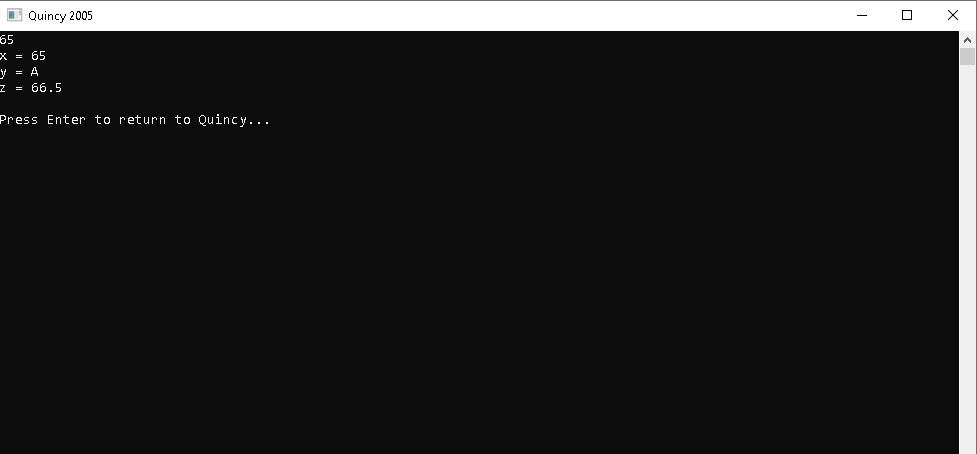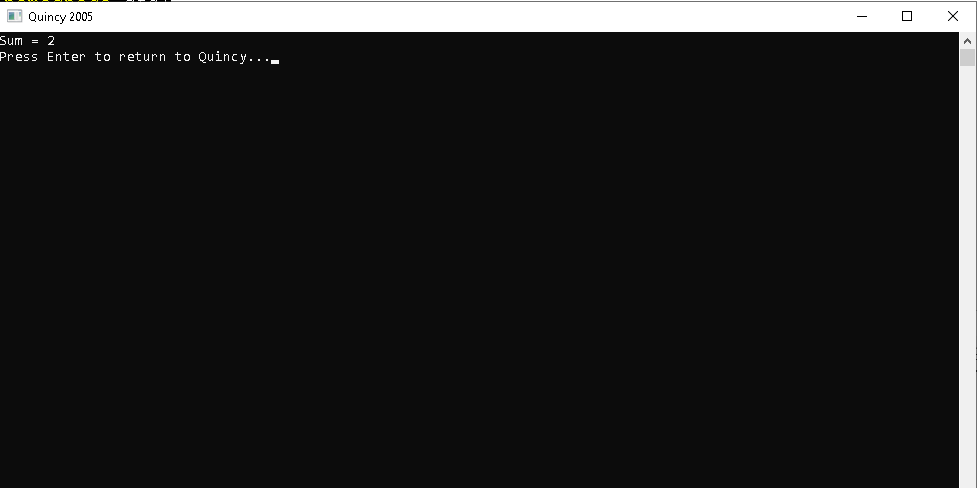# Type Conversion in C++

#### Type Conversion in C++

A cast type is essentially a conversion from one type to the next. Two kinds of conversion are available:

1.Conversion of Type Implicit Also referred to as 'automatic conversion type.'

• Done on its own using the compiler, without any external user trigger.
• Generally, more than one sort of data is present in an expression. Conversion of type (type promotion) occurs in such a situation to avoid data loss.
• All variable data types will be upgraded to the most significant data type variable.
• Signs can be lost (when signed is implicitly transformed into unsigned), and overflow can occur if the information is implicitly conversed (when long long is implicitly converted to float).

2. Explicit conversion Type: This method is also known as the casting type and is defined by the user. In this case, the user can tap the result in a particular sort of data. Two methods can be used in C++:

• Conversion via assignment: this is achieved by defining explicitly before the expression in parenthesis the required type. This might also be regarded as a strong casting.

#### Example of implicit type casting

#include <iostream> using namespace std; int main() { int x =0; // integer x char y = 'A'; // character y // y implicitly converted to int x = x + y; //0+65(ASCII value of 'A' is 65) cout<<x <<endl; // x is implicitly converted to float float z = x + 1.5; cout << "x = " << x << endl; cout << "y = " << y << endl; cout << "z = " << z << endl; return 0; }

#### OUTPUT:#### Syntax:

(type) expression

#### Example of Explicit type casting

#include <iostream> using namespace std; int main() { double x = 1.2; // Explicit type casting from double to int int add = (int)x + 1; cout << "Sum = " << add; return 0; }

#### OUTPUT:#### Upgraded to the data type of the variable with largest data type.

bool -> char -> short int -> int -> unsigned int -> long -> unsigned -> long long -> float -> double -> long double

where type specifies the type of data to which the outcome is transformed.

• Cast operator conversion: a Cast operator is a single operator, which compels one sort of information to be transformed into another.

#### C++ is supported by four casting types:

• Static Cast
• Dynamic Cast
• Const Cast
• Reinterpret Cast